Type Here to Get Search Results !

# CHEMISTRY FORM THREE TOPIC 7: CHEMICAL KINETICS, EQUILIBRIUM AND ENERGETICSThe Rate of Chemical Reactions

Copmarison between the Rates of Chemical Reactions

Compare the rates of chemical reactions

Chemical reactions take place at different rates. Some are fast whereas others are very slow. Let us consider the following reactions:

1. Addition of sodium metal to water: 2Na(s) + 2H2O(l) → 2NaOH(aq) + H2(g) The reaction takes place immediately and violently. It is therefore a fast reaction.
2. The rusting of iron in the presence of air and water giving hydrated iron (III) oxide, F2O3.XH2O: This is an extremely slow reaction.

These two reactions could be taken as representative examples of extremely fast and extremely slow reactions, respectively.There are, however, other reactions which proceed at rates intermediate between these two extremes. Rates of some of these reactions can be measured.

The rate of a chemical reaction can be measured in various ways. Let us consider the reaction between zinc and sulphuric acid to produce zinc sulphate and hydrogen gas:
Zn(s) + H2SO4(aq) → ZnSO4(aq) + H2(g)
When zinc is added to dilute sulphuric acid in a flask, they react together. The zinc slowly disappears and the gas (H2) bubbles off. After sometime, the bubbles of a gas form less quickly. The reaction is slowing down. Finally, no more bubbles appear. The reaction is over, because all the acid has been used up. Some zinc remains behind in a beaker.

In this reaction both zinc and sulphuric acid get used up in the reaction. At the same time, zinc sulphate and hydrogen form. The rate of this reaction could be determined by measuring any of the following:

• the amount of zinc used up per unit of time;
• the amount of sulphuric acid used up per unit of time;
• the amount of zinc sulphate produced per unit of time; or
• the amount of hydrogen produced per unit of time.
In general, the rate of a chemical reaction is determined by measuring the amount of reactant used up per unit of time or the amount of product produced per unit of time. Therefore, the rate of a chemical reaction simply refers to the amount of reaction which occurs in a unit time.

Experiments to Measure the Rates of Chemical Reactions

Perform experiments to measure the rates of chemical reactions

For the reaction described above, it is easiest to measure the amount of hydrogen produced per minute. The hydrogen can be collected as it bubbles off and its volume can then be measured as shown in figure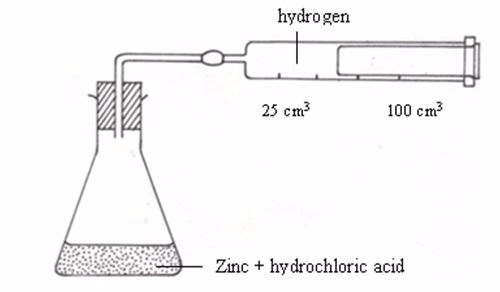Apparatus for measuring the production of gas

An experiment may be designed to measure the volume of hydrogen produced after every twenty seconds or so and then recording the data in a notebook.The table below shows sample results from such an experiment.

 Time (s) Volume of hydrogen gas (cm3) 20 13 40 22 60 30 80 37 100 41 120 44 140 46 160 47 180 47 200 47
##### Questions from the experiment

Use these data to draw a graph of time (horizontal axis) against volume of hydrogen (vertical axis).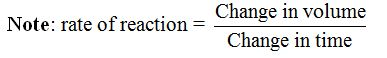There are also other ways by which rates of chemical reactions can be measured. These include measuring the:

<> change in intensity of colour: Many chemical reactions involve a change in colour. Potassium permanganate, for example, when it reacts with sulphur dioxide it changes from purple to colourless. The rate of such a reaction could be determined by measuring the rate at which the colour changes.

<> formation or disappearance of a precipitate: The reaction between hydrochloric acid and sodium thiosulphate produce a yellow precipitate of sulphur. The rate at which this precipitate forms is a measure of the rate of a reaction.

Factors Affecting the Rate of Chemical Reactions

The Effect of Concentration on the Rate of a Reaction

Describe the effect of concentration on the rate of a reaction

A chemical reaction will occur only if the particles of the reacting substances are allowed to come in contact. Increasing the concentration means increasing the density of the particles and hence the probability of particles being close together and colliding more often. Thus, a reaction can be made to go faster or slower by changing the concentration of a reactant.

The effect of concentration on the rate of reaction can be determined by mixing dilute hydrochloric acid with sodium thiosulphate solution to produce a precipitate of sulphur.Since this reaction produces a precipitate from two different colourless solutions, the intensity of the precipitate at any given moment in time represents the extent of the reaction.

The experiment to determine this effect is carried out by mixing 2M hydrochloric solution acid with 50 cm3 of the thiosulphate solution (at different concentrations as shown in the table below) and noting the time taken by the cross to disappear using a stopclock.

The procedure similar to that used to investigate the effect of temperature above is used except that, in this particular experiment, the concentration of the thiosulphate is altered each time the experiment is repeated. The following table shows the results.

The Concept of Endothermic and Exothermic Reactions

Explain the concept of endothermic and exothermic reactions

You have met many different chemical reactions so far in chemistry. But they all have one thing in common, that is, they involve an energy change. The great majority of chemical reactions are accompanied by a marked heat change.

During chemical reactions as reactants form products, there is a change in heat content. This is referred to as the enthalpy changeand is always expressed in kilojoules per mole (kJmol-1).

Two types of heat change are distinguished. Those reactions that are accompanied by evolution of heat to the surroundings are termed as exothermic reactions while those that are accompanied by absorption of heat from the surroundings are endothermic reactions.

• An exothermic reaction is one during which heat is liberated to the surroundings.
• An endothermic reaction is one during which heat is absorbed from the surroundings

When magnesium is burnt in air heat is evolved.

2Mg(s) + O2(g) → 2MgO(s)+ heat
The same case applies to the burning of coal in air.
C(s)+ O2(g) → CO2(g) + heat
Mixing sulphur nitrate and sodium chloride solutions gives a white precipitate of silver chloride and a temperature rise.
AgNO3(aq) + NaCl(aq) → AgCl(s) + NaNO3(aq)

When ammonium nitrate is dissolved in water, there is a fall in temperature. Also adding a mixture of citric acid and sodium bicarbonate to water produces bubbles and a fall in temperature. In both reactions, the temperature of the water falls because the reactions take heat energy from it. These reactions are therefore endothermic.

The heat changes that occur during any chemical reaction represent changes in the energy content of the whole system. The energy content may increase or decrease depending upon whether heat is absorbed or evolved.

Energy Level Diagrams for Exothermic and Endothermic Reactions

Draw energy level diagrams for exothermic and endothermic reactions

For exothermic reactions, the enthalpy changeis conventionally assigned a negative value. For example, when pellets of sodium hydroxide or concentrated sulphuric acid dissolve in water, heat is evolved and the system loses heat to the surrounding.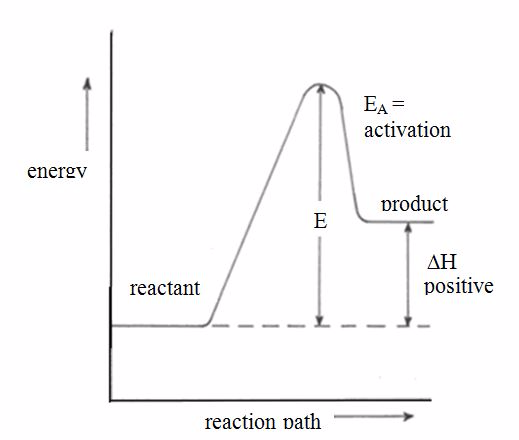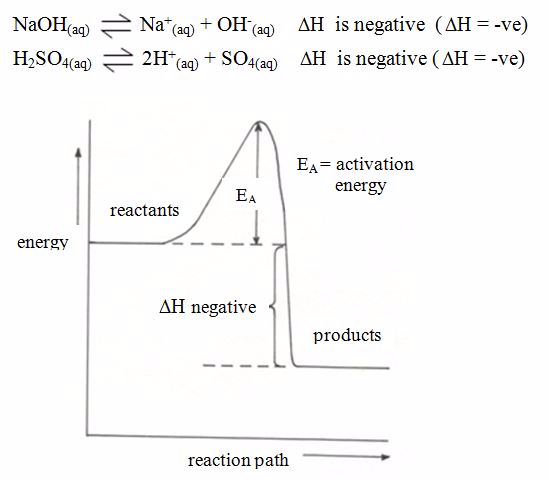Energy level diagram for exothermic reaction

For endothermic reactions, the enthalpy changeis assigned a positive value. For example, when potassium iodide or ammonium chloride dissolves in water, heat is absorbed from the surroundings.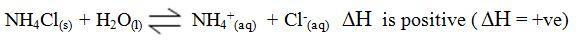Tags